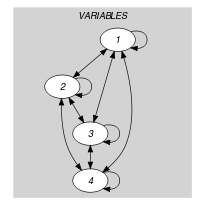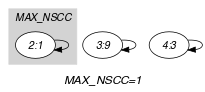## 5.294. open_alldifferent

Origin
Constraint

$\mathrm{𝚘𝚙𝚎𝚗}_\mathrm{𝚊𝚕𝚕𝚍𝚒𝚏𝚏𝚎𝚛𝚎𝚗𝚝}\left(𝚂,\mathrm{𝚅𝙰𝚁𝙸𝙰𝙱𝙻𝙴𝚂}\right)$

Synonyms

$\mathrm{𝚘𝚙𝚎𝚗}_\mathrm{𝚊𝚕𝚕𝚍𝚒𝚏𝚏}$, $\mathrm{𝚘𝚙𝚎𝚗}_\mathrm{𝚊𝚕𝚕𝚍𝚒𝚜𝚝𝚒𝚗𝚌𝚝}$, $\mathrm{𝚘𝚙𝚎𝚗}_\mathrm{𝚍𝚒𝚜𝚝𝚒𝚗𝚌𝚝}$.

Arguments
 $𝚂$ $\mathrm{𝚜𝚟𝚊𝚛}$ $\mathrm{𝚅𝙰𝚁𝙸𝙰𝙱𝙻𝙴𝚂}$ $\mathrm{𝚌𝚘𝚕𝚕𝚎𝚌𝚝𝚒𝚘𝚗}\left(\mathrm{𝚟𝚊𝚛}-\mathrm{𝚍𝚟𝚊𝚛}\right)$
Restrictions
 $𝚂\ge 1$ $𝚂\le |\mathrm{𝚅𝙰𝚁𝙸𝙰𝙱𝙻𝙴𝚂}|$ $\mathrm{𝚛𝚎𝚚𝚞𝚒𝚛𝚎𝚍}$$\left(\mathrm{𝚅𝙰𝚁𝙸𝙰𝙱𝙻𝙴𝚂},\mathrm{𝚟𝚊𝚛}\right)$
Purpose

Let $𝒱$ be the variables of the collection $\mathrm{𝚅𝙰𝚁𝙸𝙰𝙱𝙻𝙴𝚂}$ for which the corresponding position belongs to the set $𝚂$. Positions are numbered from 1. Enforce all variables of $𝒱$ to take distinct values.

Example
$\left(\left\{2,3,4\right\},〈9,1,9,3〉\right)$

The $\mathrm{𝚘𝚙𝚎𝚗}_\mathrm{𝚊𝚕𝚕𝚍𝚒𝚏𝚏𝚎𝚛𝚎𝚗𝚝}$ constraint holds since the last three (i.e., $𝚂=\left\{2,3,4\right\}$) values of the collection $〈9,1,9,3〉$ are distinct.

Typical
$|\mathrm{𝚅𝙰𝚁𝙸𝙰𝙱𝙻𝙴𝚂}|>2$
Symmetry

All occurrences of two distinct values of $\mathrm{𝚅𝙰𝚁𝙸𝙰𝙱𝙻𝙴𝚂}.\mathrm{𝚟𝚊𝚛}$ can be swapped; all occurrences of a value of $\mathrm{𝚅𝙰𝚁𝙸𝙰𝙱𝙻𝙴𝚂}.\mathrm{𝚟𝚊𝚛}$ can be renamed to any unused value.

Arg. properties

Suffix-contractible wrt. $\mathrm{𝚅𝙰𝚁𝙸𝙰𝙱𝙻𝙴𝚂}$.

Usage

In their article [HoeveRegin06], W.-J. van Hoeve and J.-C. Régin motivate the $\mathrm{𝚘𝚙𝚎𝚗}_\mathrm{𝚊𝚕𝚕𝚍𝚒𝚏𝚏𝚎𝚛𝚎𝚗𝚝}$ constraint by the following scheduling problem. Consider a set of activities (where each activity has a fixed duration 1 and a start variable) that can be processed on two factory lines such that all the activities that will be processed on a given line must be pairwise distinct. This can be modelled by using one $\mathrm{𝚘𝚙𝚎𝚗}_\mathrm{𝚊𝚕𝚕𝚍𝚒𝚏𝚏𝚎𝚛𝚎𝚗𝚝}$ constraint for each line, involving all the start variables as well as a set variable whose final value specifies the set of activities assigned to that specific factory line.

Note that this can also be directly modelled by a single $\mathrm{𝚍𝚒𝚏𝚏𝚗}$ constraint. This is done by introducing an assignment variable for each activity. The initial domain of each assignment variable consists of two values that respectively correspond to the two factory lines.

Algorithm

A slight adaptation of the flow model that handles the original $\mathrm{𝚐𝚕𝚘𝚋𝚊𝚕}_\mathrm{𝚌𝚊𝚛𝚍𝚒𝚗𝚊𝚕𝚒𝚝𝚢}$ constraint [Regin96] is described in [HoeveRegin06]. The rightmost part of Figure 3.7.28 illustrates this flow model.

generalisation: $\mathrm{𝚘𝚙𝚎𝚗}_\mathrm{𝚐𝚕𝚘𝚋𝚊𝚕}_\mathrm{𝚌𝚊𝚛𝚍𝚒𝚗𝚊𝚕𝚒𝚝𝚢}$ (control the number of occurrence of each active valueAn active value corresponds to a value occuring at a position mentionned in the set $𝚂$. with a counter variable), $\mathrm{𝚘𝚙𝚎𝚗}_\mathrm{𝚐𝚕𝚘𝚋𝚊𝚕}_\mathrm{𝚌𝚊𝚛𝚍𝚒𝚗𝚊𝚕𝚒𝚝𝚢}_\mathrm{𝚕𝚘𝚠}_\mathrm{𝚞𝚙}$ (control the number of occurrence of each active value with an interval).

Keywords
Arc input(s)

$\mathrm{𝚅𝙰𝚁𝙸𝙰𝙱𝙻𝙴𝚂}$

Arc generator
$\mathrm{𝐶𝐿𝐼𝑄𝑈𝐸}$$↦\mathrm{𝚌𝚘𝚕𝚕𝚎𝚌𝚝𝚒𝚘𝚗}\left(\mathrm{𝚟𝚊𝚛𝚒𝚊𝚋𝚕𝚎𝚜}\mathtt{1},\mathrm{𝚟𝚊𝚛𝚒𝚊𝚋𝚕𝚎𝚜}\mathtt{2}\right)$

Arc arity
Arc constraint(s)
 $•\mathrm{𝚟𝚊𝚛𝚒𝚊𝚋𝚕𝚎𝚜}\mathtt{1}.\mathrm{𝚟𝚊𝚛}=\mathrm{𝚟𝚊𝚛𝚒𝚊𝚋𝚕𝚎𝚜}\mathtt{2}.\mathrm{𝚟𝚊𝚛}$ $•$$\mathrm{𝚒𝚗}_\mathrm{𝚜𝚎𝚝}$$\left(\mathrm{𝚟𝚊𝚛𝚒𝚊𝚋𝚕𝚎𝚜}\mathtt{1}.\mathrm{𝚔𝚎𝚢},𝚂\right)$ $•$$\mathrm{𝚒𝚗}_\mathrm{𝚜𝚎𝚝}$$\left(\mathrm{𝚟𝚊𝚛𝚒𝚊𝚋𝚕𝚎𝚜}\mathtt{2}.\mathrm{𝚔𝚎𝚢},𝚂\right)$
Graph property(ies)
$\mathrm{𝐌𝐀𝐗}_\mathrm{𝐍𝐒𝐂𝐂}$$\le 1$

Graph class
$\mathrm{𝙾𝙽𝙴}_\mathrm{𝚂𝚄𝙲𝙲}$

Graph model

We generate a clique with an equality constraint between each pair of vertices (including a vertex and itself) and state that the size of the largest strongly connected component should not exceed one. Variables for which the corresponding position does not belong to the set $𝚂$ are removed from the final graph by the second and third conditions of the arc-constraint.

Parts (A) and (B) of Figure 5.294.1 respectively show the initial and final graph associated with the Example slot. Since we use the $\mathrm{𝐌𝐀𝐗}_\mathrm{𝐍𝐒𝐂𝐂}$ graph property we show one of the largest strongly connected components of the final graph. The $\mathrm{𝚘𝚙𝚎𝚗}_\mathrm{𝚊𝚕𝚕𝚍𝚒𝚏𝚏𝚎𝚛𝚎𝚗𝚝}$ holds since all the strongly connected components have at most one vertex: a value is used at most once.

##### Figure 5.294.1. Initial and final graph of the $\mathrm{𝚘𝚙𝚎𝚗}_\mathrm{𝚊𝚕𝚕𝚍𝚒𝚏𝚏𝚎𝚛𝚎𝚗𝚝}$ constraint(a) (b)